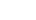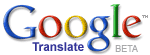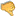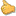ترجم الموضوع الى العربيةMathematization of PhilosophyHassan Ajami
2019 / 9 / 29

Mathematization of philosophy consists of presenting philosophical theories through the usage of mathematical equations. According to this philosophical paradigm, it is possible to accurately mathematize any philosophical theory. This is because any theory (possessing genuine content�-or-possibly true content) is a de-script-ion and/or an analysis of reality�-or-possible realities, while any actual�-or-possible reality is mathematical in nature, given that mathematical equations are the foundations of any possible reality.

For example, we could successfully mathematize Plato�s theory of knowledge. Plato says that knowledge is justified true belief. This means that there are three conditions which should be satisfied in order for us to have knowledge. These conditions are: believing, being justified in what we believe in, and the truthfulness of what we believe in. For instance, we know that people are good if and only if we believe that people are good, we are justified in believing that people are good, and our belief that people are good is true.

Plato�s theory of knowledge could be accurately presented in a mathematical equation, according to which, knowledge = believing x being justified in our belief x our belief is true (i.e. knowledge is equal to believing multiplied by justification which is in turn multiplied by true belief). This mathematical equation represents Plato�s theory of knowledge because given that knowledge = believing x being justified in our belief x our belief is true, it follows that knowledge is obtained if and only if one believes, is justified in believing, and one�s belief is true, exactly as Plato defines knowledge. This shows that we can successfully mathematize Plato�s theory of knowledge.

Moreover, the mathematical equation of Plato�s theory of knowledge entails philosophically interesting conclusions. For instance, since knowledge = believing x being justified x true belief, it follows that knowledge is a matter of degree, such that we could know more�-or-less. If knowledge = believing x being justified x true belief, then when believing, being justified, and the probability of having a true belief are maximized, knowledge is maximized, otherwise it is minimized. This implies that knowledge is a matter of degree.

Plato�s theory of Forms could also be presented in a mathematical equation. According to Plato, the Forms exist in a realm�-or-universe separate from and independent of our material universe, such that the material objects are as they are in virtue of participating in the Forms, given that the Forms are the real existents. For example, all of us are good because we participate in the Form of goodness. Plato�s theory could be mathematized in the following way: our universe = matter + participation in the Forms. It follows from this mathematical equation that participation in the Forms = our universe � matter (i.e. participation in the Forms is equal to our universe minus matter). Hence, participation in the Forms and thus the Forms themselves are separate from and independent of matter, leading to Plato�s account that the Forms exist in a realm independent of and separate from our material universe. The success of this mathematical equation in expressing Plato�s theory of Forms shows that it is an accurate representation of Plato�s account, and hence we can successfully mathematize his theory.

Yet Aristotle rejects Plato�s theory that the Forms exist independently of and separate from our material universe. Instead, for Aristotle, the forms exist in our material universe. Aristotle�s theory could also be mathematized through presenting it in the following mathematical equation: our universe = matter x forms (i.e. our universe is equal matter multiplied by forms). It mathematically follows from this equation that our universe divided by matter is equal to the forms, and our universe divided by the forms is equal to matter. Therefore, forms could not exist independently of and separate from matter, exactly as matter could not exist without forms, otherwise our universe becomes indefinite (given that whatever is divided by zero, such as zero matter�-or-zero forms, is indefinite). This shows that this mathematical equation (i.e. our universe = matter x forms) is successful in presenting Aristotle�s theory. And all of these examples of the mathematization of the previous philosophical theories prove that we could successfully mathematize any philosophical theory, leading to the conclusion that mathematization of philosophy is possible.Bad 12345678910 Very good